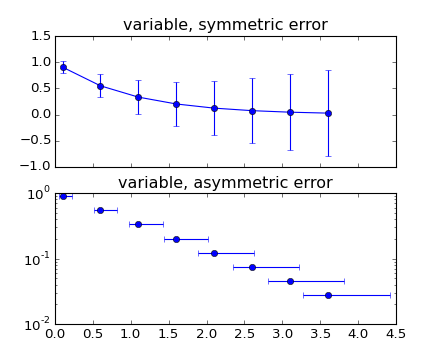### We're updating the default styles for Matplotlib 2.0

Learn what to expect in the new updates#### Previous topic

statistics example code: errorbar_demo.py

#### Next topic

statistics example code: errorbar_limits.py

# statistics example code: errorbar_demo_features.py¶```"""
Demo of errorbar function with different ways of specifying error bars.

Errors can be specified as a constant value (as shown in `errorbar_demo.py`),
or as demonstrated in this example, they can be specified by an N x 1 or 2 x N,
where N is the number of data points.

N x 1:
Error varies for each point, but the error values are symmetric (i.e. the
lower and upper values are equal).

2 x N:
Error varies for each point, and the lower and upper limits (in that order)
are different (asymmetric case)

In addition, this example demonstrates how to use log scale with errorbar.
"""
import numpy as np
import matplotlib.pyplot as plt

# example data
x = np.arange(0.1, 4, 0.5)
y = np.exp(-x)
# example error bar values that vary with x-position
error = 0.1 + 0.2 * x
# error bar values w/ different -/+ errors
lower_error = 0.4 * error
upper_error = error
asymmetric_error = [lower_error, upper_error]

fig, (ax0, ax1) = plt.subplots(nrows=2, sharex=True)
ax0.errorbar(x, y, yerr=error, fmt='-o')
ax0.set_title('variable, symmetric error')

ax1.errorbar(x, y, xerr=asymmetric_error, fmt='o')
ax1.set_title('variable, asymmetric error')
ax1.set_yscale('log')
plt.show()
```

Keywords: python, matplotlib, pylab, example, codex (see Search examples)